# 4 Dimensional Keller Graph

The Second DIMACS Implementation Challenge: 1992-1993

Originator: Panos Pardalos

NP Hard Problems: Maximum Clique, Graph Coloring, and Satisfiability, The Second DIMACS Implementation Challenge: 1992-1993.

Graphs based on Keller's conjecture on tilings using hypercubes.

## Examples

### Basic Examples

Retrieve the graph:

 In:=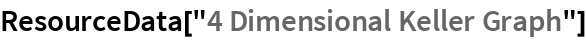Out=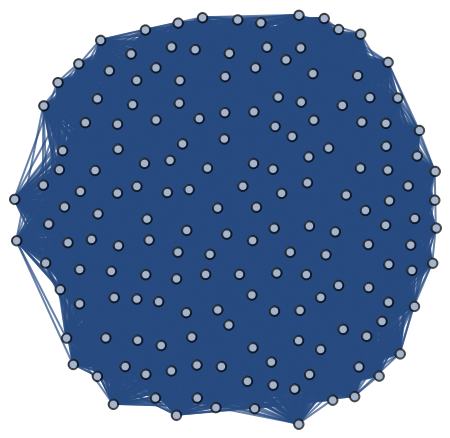Summary properties:

 In:=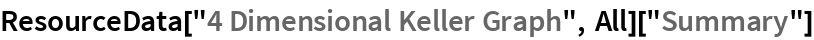Out=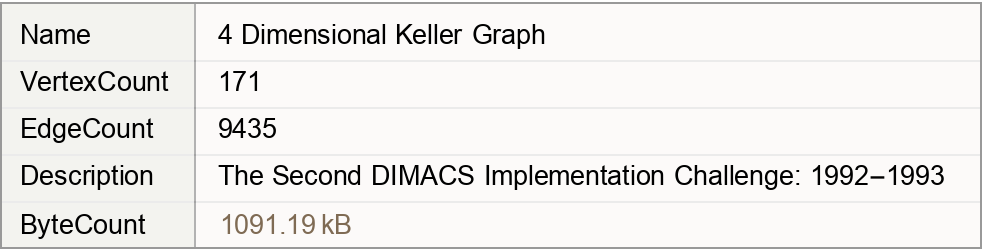### Basic Applications

Find the maximum clique:

 In:=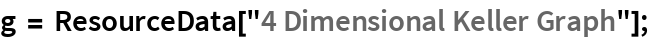In:=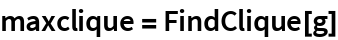Out=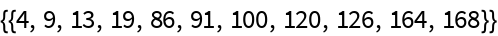Show the maximum clique:

 In:=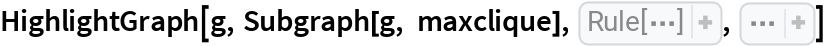Out=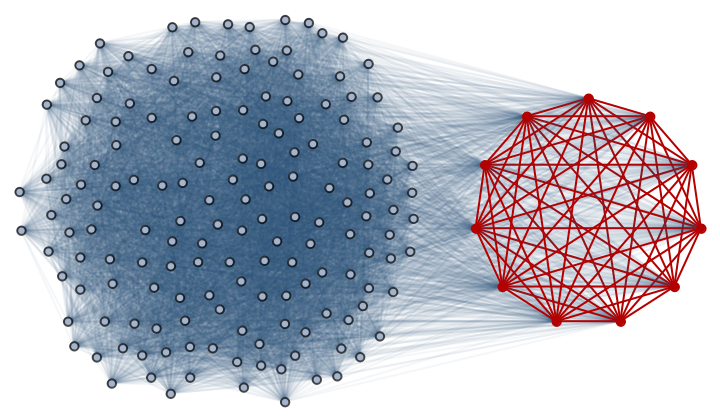Wolfram Research, "4 Dimensional Keller Graph" from the Wolfram Data Repository (2019)